# June 3rd Electronics TV's & Laptops

### Auction Details

Electronics TV's & Laptops
Choose Your Layout >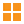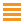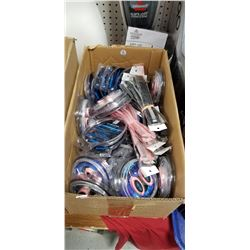101 - Shelf One
CDC Auctions
Shelf One
Bidding Has Concluded

Sold to f***r for (20.00 + 2.60) x 1 = 22.60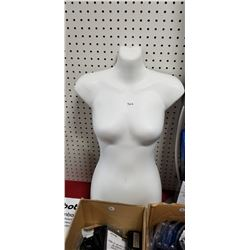102 - Shelf One
CDC Auctions
Shelf One
Bidding Has Concluded

Sold to f***r for (10.00 + 1.30) x 1 = 11.30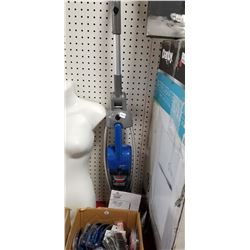103 - Shelf One
CDC Auctions
Shelf One
Bidding Has Concluded

Sold to f***r for (15.00 + 1.95) x 1 = 16.95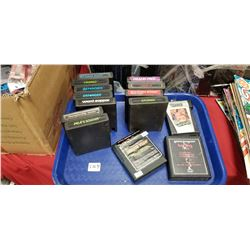104 - Shelf One
CDC Auctions
Shelf One
Bidding Has Concluded

Sold to O********c.. for (10.00 + 1.30) x 1 = 11.30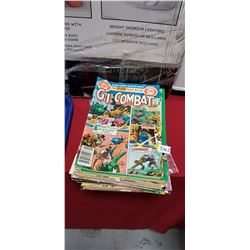105 - Shelf One
CDC Auctions
Shelf One
Bidding Has Concluded

Sold to O********c.. for (20.00 + 2.60) x 1 = 22.60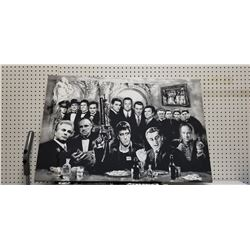106 - Shelf Two
CDC Auctions
Shelf Two
Bidding Has Concluded

Sold to f***r for (15.00 + 1.95) x 1 = 16.95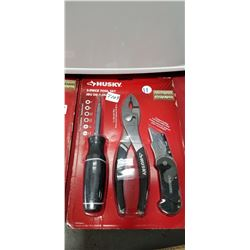109 - Shelf One
CDC Auctions
Shelf One
Bidding Has Concluded

Sold to f***r for (10.00 + 1.30) x 1 = 11.30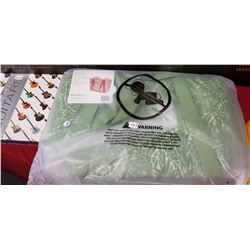113 - Rachel Ray Bag
CDC Auctions
Shelf One
Bidding Has Concluded

Sold to f***r for (10.00 + 1.30) x 1 = 11.30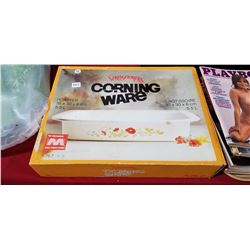115 - Shelf One
CDC Auctions
Shelf One
Bidding Has Concluded

Sold to f***r for (10.00 + 1.30) x 1 = 11.30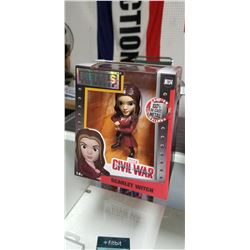116 - Shelf One
CDC Auctions
Shelf One
Bidding Has Concluded

Sold to O********c.. for (10.00 + 1.30) x 1 = 11.30117 - Shelf One
CDC Auctions
Shelf One
Bidding Has Concluded

Sold to O********c.. for (10.00 + 1.30) x 1 = 11.30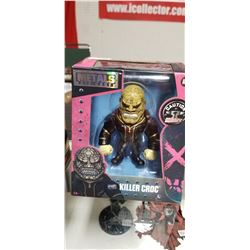118 - Shelf One
CDC Auctions
Shelf One
Bidding Has Concluded

Sold to O********c.. for (10.00 + 1.30) x 1 = 11.30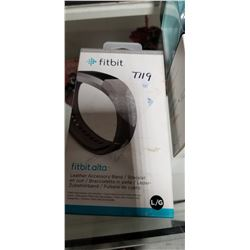119 - Shelf One
CDC Auctions
Shelf One
Bidding Has Concluded

Sold to R*****g for (17.50 + 2.28) x 1 = 19.78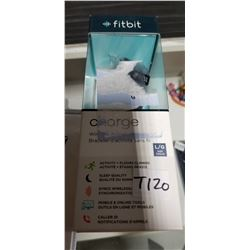120 - Fitbit Charge
CDC Auctions
Shelf One
Bidding Has Concluded

Sold to d*******M for (40.00 + 5.20) x 1 = 45.20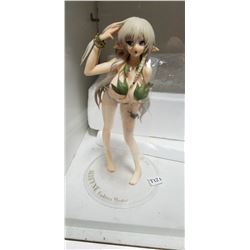121 - Shelf One
CDC Auctions
Shelf One
Bidding Has Concluded

Sold to J********s for (22.50 + 2.93) x 1 = 25.43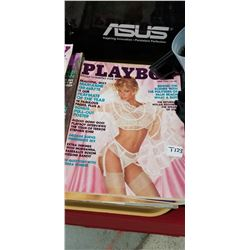123 - Shelf One
CDC Auctions
Shelf One
Bidding Has Concluded

Sold to f***r for (10.00 + 1.30) x 1 = 11.30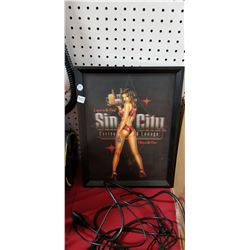127 - Shelf One
CDC Auctions
Shelf One
Bidding Has Concluded

Sold to f***r for (20.00 + 2.60) x 1 = 22.60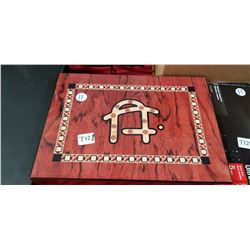128 - Shelf One
CDC Auctions
Shelf One
Bidding Has Concluded

Sold to f***r for (15.00 + 1.95) x 1 = 16.95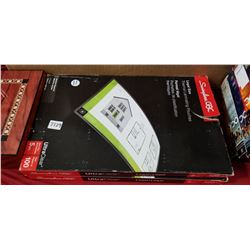129 - Shelf One
CDC Auctions
Shelf One
Bidding Has Concluded

Sold to f***r for (10.00 + 1.30) x 1 = 11.30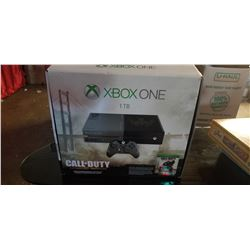133 - X Box One - 1TB
CDC Auctions
Shelf One
Bidding Has Concluded

Sold to f***r for (180.00 + 23.40) x 1 = 203.40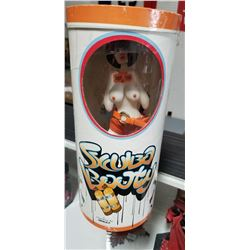134 - Shelf One
CDC Auctions
Shelf One
Bidding Has Concluded

Sold to f***r for (10.00 + 1.30) x 1 = 11.30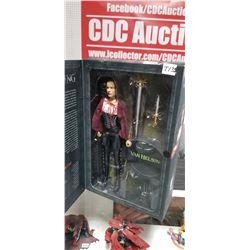135 - Shelf One
CDC Auctions
Shelf One
Bidding Has Concluded

Sold to f***r for (15.00 + 1.95) x 1 = 16.95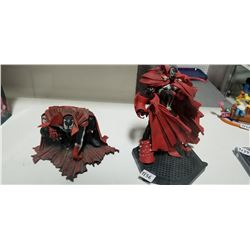136 - Shelf One
CDC Auctions
Shelf One
Bidding Has Concluded

Sold to f***r for (22.50 + 2.93) x 1 = 25.43137 - Shelf One
CDC Auctions
Shelf One
Bidding Has Concluded

Sold to f***r for (7.50 + 0.98) x 1 = 8.48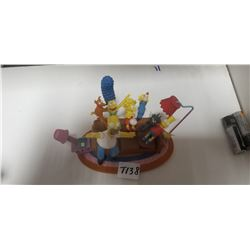138 - Shelf One
CDC Auctions
Shelf One
Bidding Has Concluded

Sold to f***r for (17.50 + 2.28) x 1 = 19.78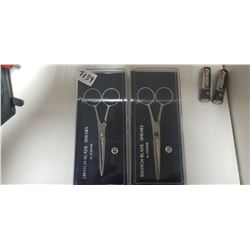139 - Shelf One
CDC Auctions
Shelf One
Bidding Has Concluded

Sold to f***r for (15.00 + 1.95) x 1 = 16.95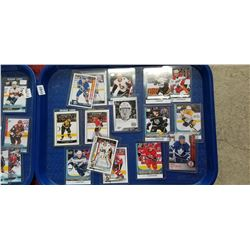141 - Shelf One
CDC Auctions
Shelf One
Bidding Has Concluded

Sold to f***r for (25.00 + 3.25) x 1 = 28.25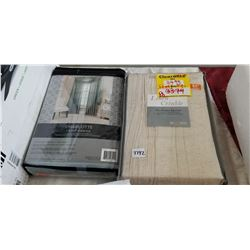142 - Shelf Two
CDC Auctions
Shelf Two
Bidding Has Concluded

Sold to f***r for (10.00 + 1.30) x 1 = 11.30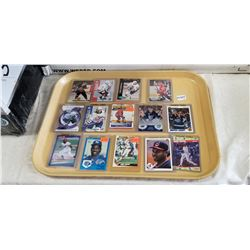145 - Shelf One
CDC Auctions
Shelf One
Bidding Has Concluded

Sold to f***r for (12.50 + 1.63) x 1 = 14.13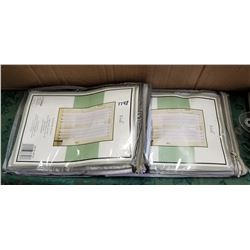148 - Shelf One
CDC Auctions
Shelf One
Bidding Has Concluded

Sold to f***r for (10.00 + 1.30) x 1 = 11.30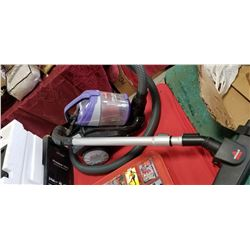150 - Shelf One
CDC Auctions
Shelf One
Bidding Has Concluded

Sold to f***r for (10.00 + 1.30) x 1 = 11.30